Get instant live expert help with Excel or Google Sheets“My Excelchat expert helped me in less than 20 minutes, saving me what would have been 5 hours of work!”

#### Post your problem and you’ll get expert help in seconds.

Your message must be at least 40 characters
Our professional experts are available now. Your privacy is guaranteed.

# Here’s a Guide on Finding the Median in a Pivot Table

We can use a formula that combines the MEDIAN and IF functions to find the median of a set of data if the values meet a criteria in a Pivot Table. The steps below will walk through the process.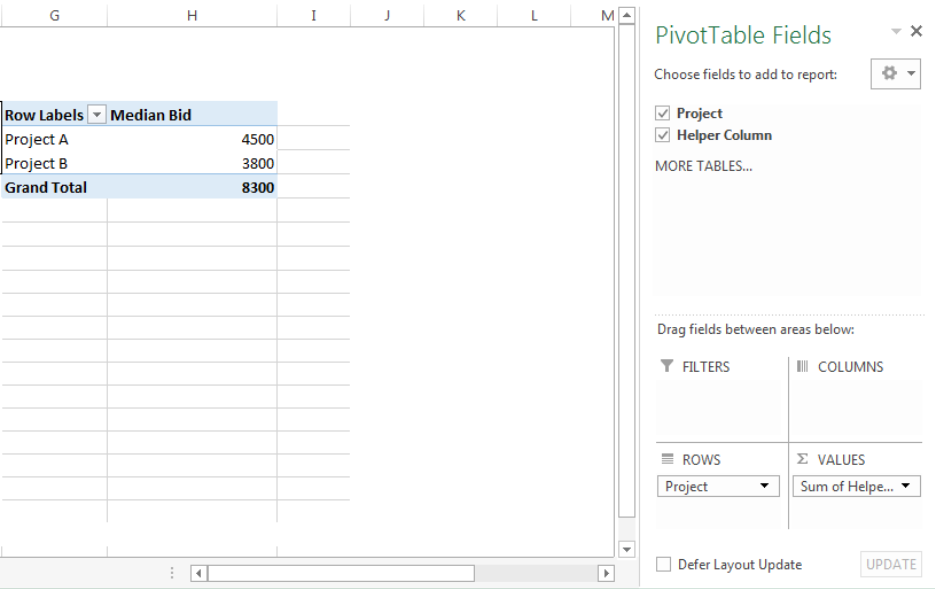Figure 1- How to Find the Median in a Pivot Table

## Syntax

`=MEDIAN(IF(logical_test,value_if_true,value_if_false))`

• logical_test: This is the criteria that must be met for the median function to operate
• value_if_true: This is the returned result by the IF Function that tells the median function that the criteria is met and the median function can operate
• value_if_false: This is the opposite of value_if_true

## Formula

`=MEDIAN(IF(\$B\$5:\$B\$10=E5,\$C\$5:\$C\$10))`

## Setting up the Data

Contractors have placed their bids for two separate projects in a company. The manager intends to find the median bid for Project A and Project B with a Pivot Table

• We will insert the Project names and Bids in Column B and Column C respectively
• Pivot Tables do possess the MEDIAN Function, hence, we have created a helper column in Column F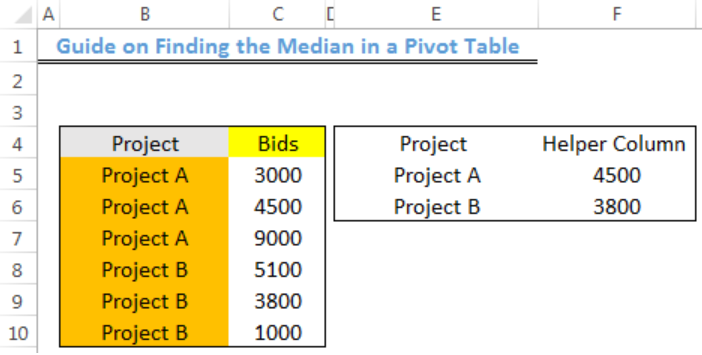Figure 2 – Setting up the Data

## How to Create the Helper Column

• We will click on Cell F5
• We will insert the formula below into Cell F5
`=MEDIAN(IF(\$B\$5:\$B\$10=\$E\$5,\$C\$5:\$C\$10))`
• Because this is an array formula, we will press CTRL + SHIFT + ENTER
• We will click on Cell F5 again
• We will double click on the fill handle tool which is the small plus sign you see at the bottom right of Cell F5. Select and drag down to copy the formula to Cell F6.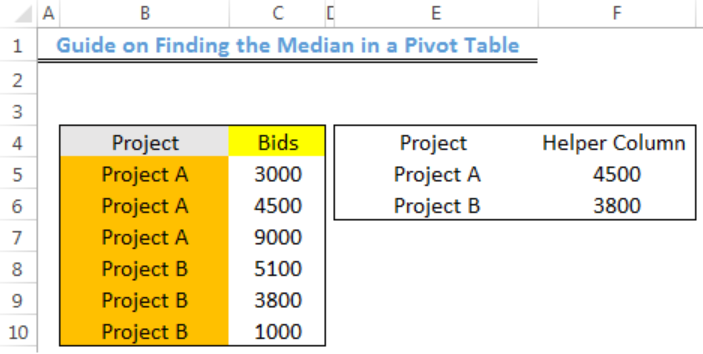Figure 3- Creating the MEDIANIF Helper Column

## Creating the Pivot Table

• We will select the range (E4:F6) of the table containing the helper column
• We will click on Cell G4 where our PIVOT TABLE will be returned in the existing worksheet
• We will click on the Insert tab and click on Pivot TableFigure 4- Clicking on Pivot Table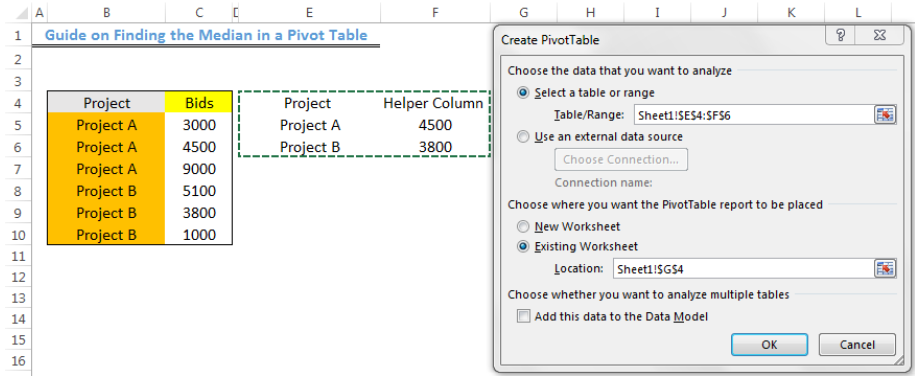Figure 5- Creating the Pivot Table

• We will press OK
• We will check the PROJECT and HELPER COLUMN boxes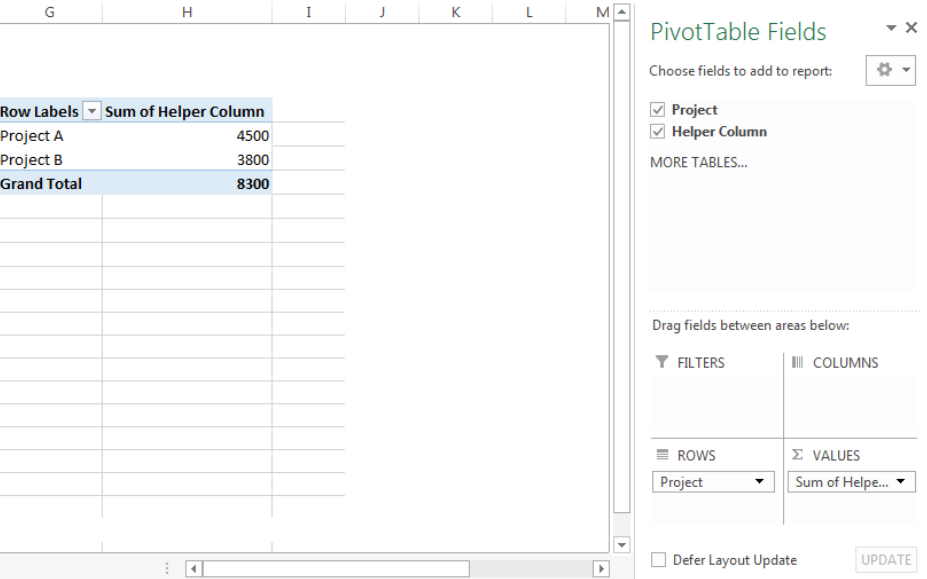Figure 6- Checking the Project and Helper Column boxes

• We will click on SUM OF HELPER COLUMN and change it to Median Bid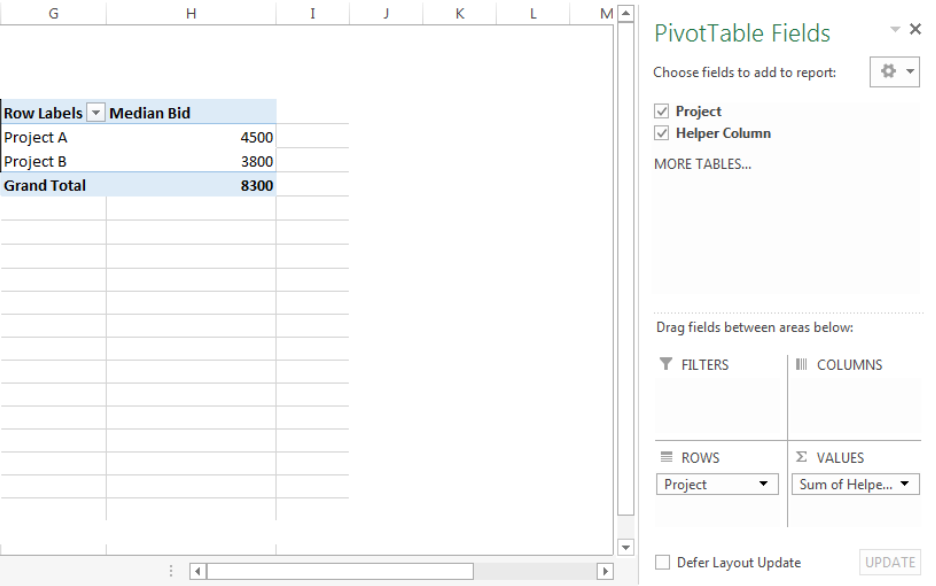Figure 7- Median in a Pivot Table

## Explanation

`=MEDIAN(IF(\$B\$5:\$B\$10=E5,\$C\$5:\$C\$10))`

In this formula, the IF function checks the range (B5:B10) for cells that have values equal to E5 (Project A). The identified cells are matched with the corresponding cell in Column C and this is returned to the MEDIAN function.

The MEDIAN function returns the middle number for an odd number of values and averages the two middle numbers for an even number of values as the result.

## Instant Connection to an Expert through our Excelchat Service

Most of the time, the problem you will need to solve will be more complex than a simple application of a formula or function. If you want to save hours of research and frustration, try our live Excelchat service! Our Excel Experts are available 24/7 to answer any Excel question you may have. We guarantee a connection within 30 seconds and a customized solution within 20 minutes.

Are you still looking for help with the Average function? View our comprehensive round-up of Average function tutorials here.

Solution examplesI need to convert the sum of 3 cells into a single number, ie if cell a and b = 200, 150 and cell c = 0, i only want cells with a value greater than zero to be counted until such a time that cell gets a value
Solved by V. L. in 20 minsHi, I am trying to get a sum of three separate cells in excel by typing "=H45+H23+H37"...and eventhough only one of the cells shows "0.00", and the remaining two cells have positive balance, the result I get is "0.00".................
Solved by K. L. in 18 minsHi , I have two columns in XLS and it has value . I need to check if either of the two value is true along with third column which has value . I need to count such columns
Solved by Z. D. in 40 minsi want to sum columns c thru k into column l in first line then automatically sum successive columns and lines in columns c thru k as numbers are added so that column l shows running total automatically.
Solved by M. H. in 39 minsHello, I need help regarding indirect formula of excel. 1. What is the purpose of using this formula? 2. If we use this formula with sum formula, then why we are using this with sum formula, we can also perform addition from different sheets without indirect formula ?
Solved by O. D. in 21 mins